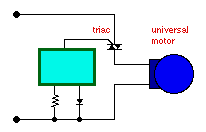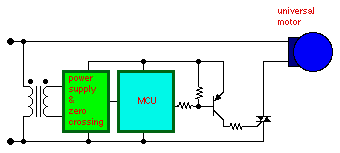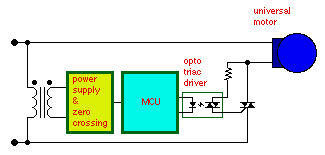• Introduction
• What is in this manual
• What is Caspoc
• User interface
• Introduction
• Starting
• Simulation
• Editing
• Viewing and printing
• Getting Started
• Basic editing
• Simulation in the time domain
• Basic User Interface Topics
• Editing
• Simulation
• Viewing
• Library
• Reports
• Project management
• Circuit and Block Diagram Components
• Introduction
• Cscript and user defined functions
• Component parameters
• Modeling Topics
• Introduction
• Power Electronics
• Semiconductors
• Electrical Machines
• Electrical drives
• Power Systems
• Mechanical Systems
• Thermal Systems
• Magnetic Circuits
• Green Energy
• Coupling to FEM
• Experimenter
• Analog hardware description language
• Embedded C code Export
• Coupling to Spice
• Small Signal Analysis
• Matlab coupling
• Tips and tricks
• Appendices

## Phase Angle ControlClick to Close this View Phase Angle control uses a low frequency switch to chop an AC sine wave. The firing angle of the switch is varied. The average voltage will be proportional the area under the sine wave. Thus, the average voltage is the integral from the firing angle to the zero crossing, the cosine of the firing angle. Phase Angle control provides a very inexpensive method to control the average voltage of an AC source. However, the low frequency ac waveform presented to the motor will create some torque ripple and acoustic noise. All universal motors operated from an AC source will have some torque ripple. Also, the abrupt switching characteristics of the thyristors creates a great deal of electrical noise.Click to Close this View Permanent magnet DC motors require a rectified dc source. Two SCRs and two diodes are often used to control the voltage of a dc bus. This movie illustrated the principle. If a large capacitor is used the output will be a dc voltage proportional to the sine of the firing angle. This method only requires varying the firing angle by 45�.

## Phase Angle Control TopologiesA very simply off line motor drive can be built using a Triac and a control IC. This circuit can control the speed of a universal motor. A universal motor is a series wound DC motor. The circuit uses phase angle control to vary the effective motor voltage.A micro controller can also be used to control a Triac. A PNP may be used to drive the Triac. As shown, the MCU ground is connected to the AC line. The gate trigger current is lower if instead the MCU 5V supply is connected to the AC line.

The MCU must have some means of detecting zero crossings and a timer that can control the Triac firing. A general purpose timer with one input capture and one output compare makes an ideal phase angle control.In some cases it is necessary to isolate the MCU from the AC line for safety or due to electrical noise susceptibility. An opto-Triac driver can be used to drive a large Triac. Small motors may be driven directly by an opto-Triac.